Author Message
 Fleet Admiral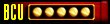Age: 22 Zodiac: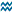Joined: 26 Nov 2002 Posts: 11439 Location: Netherlands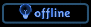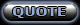Posted: 04-15-2006 10:00 PM Post subject: How to detect and fix problematic ship plugins

BCMP has a slight problem when it comes to ships with spaces in their name.
This thread is about the problems that arise when BCMP creates a ship plugin for such a ship. It will result in a BSOD.
This thread will guide you through on how to see that it is a ship plugin related problem and how to fix it.

First a rule in this thread:

• Only post faulty ship plugins here, _no_ discussion, any other questions may be put forward through PM, e-mail or MSN. _NOT_ in this thread.

I like to see the following structure in this thread:
One person posts a plugin.
I post after it with the corrected one.

For the record, I'm going to describe _one_ possible problem. It's the most common problem though. Other problems will most likely not even get to the end user because those will be tested by the creators of the plugin. If you think (or hopefully, know) it's a faulty ship plugin, put it up and clearly (make your post yellow or something) mark you post that you think (or hopefully, know) it's a faulty ship plugin, but not a BCMP caused problem. I'll get back to you as soon as possible.

Identifying the problem
The symptom of the problem will be the BSOD in whatever form it might be.
But if you look closer to the console output, then you will see that it refers to Foundation, and another file. In the end, it says it has gotten a syntax error.
In the following screenshot, you will see the phrases:
 Quote: File .\Scripts\Foundation.py, line 645, in LoadExtraShips

 Quote: SyntaxError: invalid syntax

And lastly, something similar to this (it goes before the SyntaxError one):
 Quote: File .\Scripts\Custom\Ships\Galaxy Escape Pod.py, line 38 fffFoundation.ShipDef.Galaxy Escape Pod = Foundation.FedShipDef(abbrev, species, {name: longName, iconName: iconName, shipFile: shipFile })Numbers and names may be different, and more errors might be before or after the one I described here.

The actual problem
Before you jump to conclusions, the filename isn't the problem, it can be anything, even Chinese (though I wouldn't really recommend it:P).

For starters, it's: "Foundation.ShipDef.Galaxy Escape Pod".
As soon as you fix that, you will run in a couple more problems, that are entirely related to this.

The problem here is that it's using spaces in the name, it's rather logical to assume that in a programming language (such as Python, as BC is) spaces in variables name's aren't a good idea, how is it going to see what a variable name is and what isn't, or more importantly, where does it end and where does a possible operator or something else start?

How to fix it, remove the spaces.
The best example would be to give you 2 pieces of code (from the plugin the image refers to) one in which it's faulty, one in which it's correct. The problematic changes and fixes will be marked.

The faulty one:
 Quote: Foundation.ShipDef.Galaxy Escape Pod = Foundation.FedShipDef(abbrev, species, { 'name': longName, 'iconName': iconName, 'shipFile': shipFile }) #ffffffffffffffffffffffffffffffffffffffffffffffffffffffffffffffffffffffffffffffffffff*# ######################################## #ffffffffffffffffffffffffffffffffffffffffffffffffffffffffffffffffffffffffffffffffffff*# # Uncomment these if you have TGLffffffffffffffffffffffffffffffffff*# #ffffffffffffffffffffffffffffffffffffffffffffffffffffffffffffffffffffffffffffffffffff*# # Foundation.ShipDef.Galaxy Escape Pod.hasTGLName = 1 # Foundation.ShipDef.Galaxy Escape Pod.hasTGLDesc = 1 #ffffffffffffffffffffffffffffffffffffffffffffffffffffffffffffffffffffffffffffffffffff*# # Otherwise, uncomment this and type something in:ffffffffffff# #ffffffffffffffffffffffffffffffffffffffffffffffffffffffffffffffffffffffffffffffffffff*# Foundation.ShipDef.Galaxy Escape Pod.desc = 'No Description Available' #ffffffffffffffffffffffffffffffffffffffffffffffffffffffffffffffffffffffffffffffffffff*# ######################################## #ffffffffffffffffffffffffffffffffffffffffffffffffffffffffffffffffffffffffffffffffffff*# # These register the ship with the QuickBattle menus. Don't touch them!!! # #ffffffffffffffffffffffffffffffffffffffffffffffffffffffffffffffffffffffffffffffffffff*# if menuGroup:fffffffffffFoundation.ShipDef.Galaxy Escape Pod.RegisterQBShipMenu(menuGroup) if playerMenuGroup:fffffFoundation.ShipDef.Galaxy Escape Pod.RegisterQBPlayerShipMenu(playerMenuGroup) if Foundation.shipList._keyList.has_key(longName): fffffFoundation.ShipDef.__dict__[longName].friendlyDetails = Foundation.shipList[longName].friendlyDetails fffffFoundation.ShipDef.__dict__[longName].enemyDetails = Foundation.shipList[longName].enemyDetails #ffffffffffffffffffffffffffffffffffffffffffffffffffffffffffffffffffffffffffffffffffff*# ########################################

Now, at the end, the .has_key(longName) things might seem correct, but the fix will make that invalid, that's why it's also been made bold (it's part of those couple of more problems I mentioned earlier)

The corrected one:
 Quote: Foundation.ShipDef.GalaxyEscapePod = Foundation.FedShipDef(abbrev, species, { 'name': longName, 'iconName': iconName, 'shipFile': shipFile }) #ffffffffffffffffffffffffffffffffffffffffffffffffffffffffffffffffffffffffffffffffffff*# ######################################## #ffffffffffffffffffffffffffffffffffffffffffffffffffffffffffffffffffffffffffffffffffff*# # Uncomment these if you have TGLffffffffffffffffffffffffffffffffff*# #ffffffffffffffffffffffffffffffffffffffffffffffffffffffffffffffffffffffffffffffffffff*# # Foundation.ShipDef.GalaxyEscapePod.hasTGLName = 1 # Foundation.ShipDef.GalaxyEscapePod.hasTGLDesc = 1 #ffffffffffffffffffffffffffffffffffffffffffffffffffffffffffffffffffffffffffffffffffff*# # Otherwise, uncomment this and type something in:ffffffffffff# #ffffffffffffffffffffffffffffffffffffffffffffffffffffffffffffffffffffffffffffffffffff*# Foundation.ShipDef.GalaxyEscapePod.desc = 'No Description Available' #ffffffffffffffffffffffffffffffffffffffffffffffffffffffffffffffffffffffffffffffffffff*# ######################################## #ffffffffffffffffffffffffffffffffffffffffffffffffffffffffffffffffffffffffffffffffffff*# # These register the ship with the QuickBattle menus. Don't touch them!!! # #ffffffffffffffffffffffffffffffffffffffffffffffffffffffffffffffffffffffffffffffffffff*# if menuGroup:fffffffffffFoundation.ShipDef.GalaxyEscapePod.RegisterQBShipMenu(menuGroup) if playerMenuGroup:fffffFoundation.ShipDef.GalaxyEscapePod.RegisterQBPlayerShipMenu(playerMenuGroup) if Foundation.shipList._keyList.has_key(GalaxyEscapePod): fffffFoundation.ShipDef.__dict__[GalaxyEscapePod].friendlyDetails = Foundation.shipList[GalaxyEscapePod].friendlyDetails fffffFoundation.ShipDef.__dict__[GalaxyEscapePod].enemyDetails = Foundation.shipList[GalaxyEscapePod].enemyDetails #ffffffffffffffffffffffffffffffffffffffffffffffffffffffffffffffffffffffffffffffffffff*# ########################################

That's it.
I've also marked parts that are commented out, but there are plugins that don't have them commented.

If you can't fix it, put up the faulty plugin here and I'll fix it.

_________________How to beat the Black Screen Of Death and Script problems Also handles Console/Screen/Error report/dump.
BC Technical FAQ [Last updated 26/12/2005]
"Trust me, I'm a con artist" - Moist von Lipwig, "Going Postal, Discworld"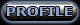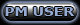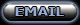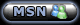Display posts from previous: All Posts1 Day7 Days2 Weeks1 Month3 Months6 Months1 Year Oldest FirstNewest First
 All times are GMTPage 1 of 1

 Jump to: Select a forum BC Forums----------------BC ModdingBC ScriptingBC Bridge ModdingBC DownloadsBC MultiplayerBC GeneralNanoFX GE ProjectBC2 ProjectTechnical SupportGamehack Magazine General Forums----------------Forum AnnouncementsRole Playing ForumGraphics Art ForumGeneral DiscussionSuggestionsStar Trek Tech Talk Adult Forums----------------Rants & RavesPolitical DebateSpam Only
You cannot post new topics in this forum
You cannot reply to topics in this forum
You cannot edit your posts in this forum
You cannot delete your posts in this forum
You cannot vote in polls in this forum